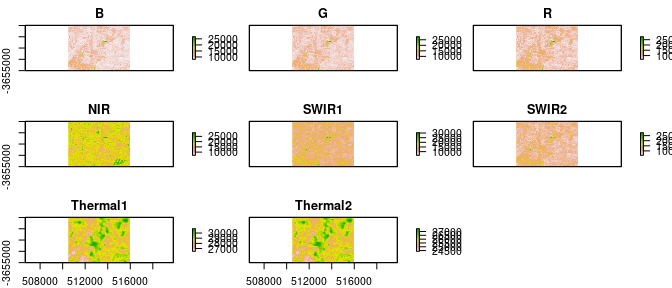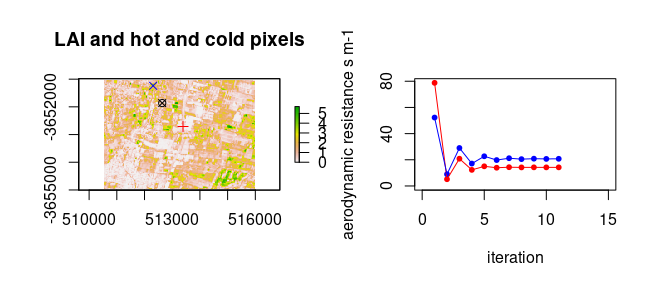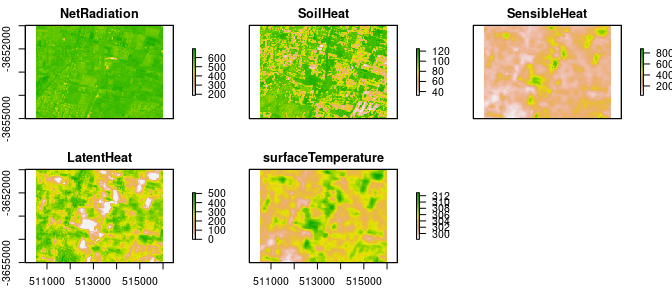# Using Landsat 8 data with METRIC and water package

#### 2018-04-24

Landsat 8 has better radiometric resolution, a two bands in the thermal region. In water package we do not provide a method for the estimation of surface reflectace. However, USGS offers surface reflectance processed trought ESPA. water package uses ESPA products when running METRIC algorithm with Landsat 8 data. In this vignette we describe how to use Landsat 8 data with METRIC and water package.

## Differences between Landsat 7 and 8

Landsat 8 has two new spectral bands: a deep blue visible channel (band 1) specifically designed for water resources and coastal zone investigation, and a new infrared channel (band 9) for the detection of cirrus clouds. Also, the TIRS instrument collects two spectral bands for the wavelength covered by a single band on the previous TM and ETM+ sensors. The two bands from TIRS allows to use the split windows method for the estimation of land surface temperature. The estimation of surface reflectance is not included in water package. Nevertheless, water package reads the surface reflectance products obtained from ESPA. Finally, as the band limits differ from the ones in Landsat 7, the estimation of albedo needs a new set of coefficients. In water we include coefficients estimated using SMARTS. In the next section we show how to estimate METRIC model with Landsat 8 data using the simple procedure.

## Simple procedure

As Landsat scenes are almost 1Gb in size, we do not recommend using one complete scene without cropping an area-of-interest first. An area-of-interest can be defined using createAoi() function:

library(water)
aoi <- createAoi(topleft = c(500000, -3644000), bottomright = c(526000, -3660000))

To be able to run METRIC with Landsat 8 data, the ESPA surface reflectance products must be available in the working directory. If we list the contents of the L8 example files provided with water, we can see we have 38 files: 2 metadata files, the original and the one from ESPA and 14 tif files with the raw data. The Landsat scene can loaded using loadImage() function. And the SR data with loadImage.SR:

raw_data_folder <- system.file("extdata", package="water")
image <- loadImage(path=raw_data_folder, aoi=aoi, sat="L8")
image.SR <- loadImageSR(path=raw_data_folder, aoi=aoi)
plot(image)Finally, the simple procedure for estimating METRIC can be applied to the image:

csvfile <- system.file("extdata", "INTA.csv", package="water")
MTLfile <- system.file("extdata", "LC82320832016040LGN00_MTL.txt", package="water")
WeatherStation <- read.WSdata(WSdata = csvfile,
datetime.format =  "%Y/%m/%d %H:%M",
columns=c("date" = 1, "time" = 1, "radiation" = 5,
"wind" = 6, "RH" = 3, "temp" = 2, "rain" = 4),
lat=-33.00513, long= -68.86469, elev=927, height= 2,
MTL=MTLfile)
Energy.Balance <- METRIC.EB(image.DN = image, image.SR = image.SR,
plain=TRUE, aoi=aoi, n = 5, WeatherStation = WeatherStation,
ETp.coef = 1.2, sat="L8", alb.coeff = "Olmedo", LST.method = "SW",
LAI.method = "metric2010", Z.om.ws = 0.03, MTL = MTLfile)
##   pixel      X        Y       Ts  LAI NDVI type
## 1 10585 513390 -3652710 309.7776 0.05 0.19  hot
## 2  1533 512310 -3651240 302.7335 3.32 0.71 coldplot(Energy.Balance\$EB)